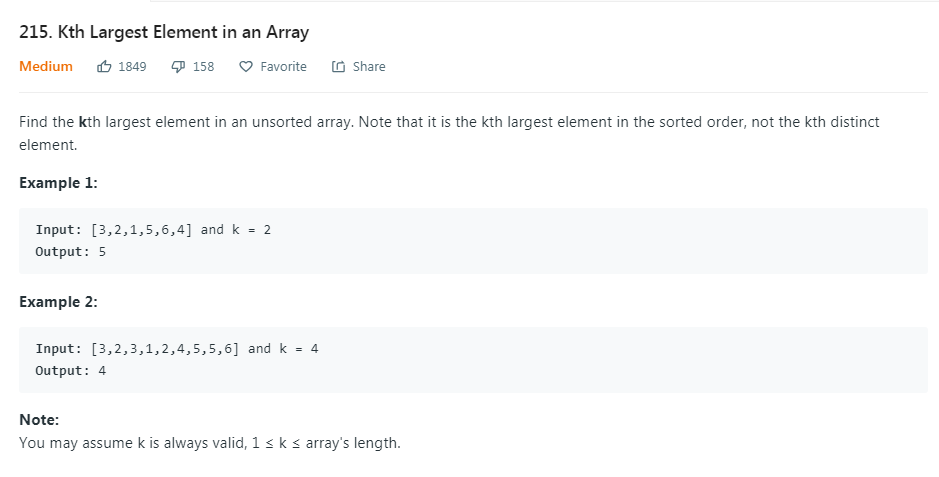## LeetCode 215 Kth Largest Element in an Array``````class Solution {
public int findKthLargest(int[] nums, int k) {
int[] temp=new int[k];
Arrays.sort(nums,0,k);
for(int i=0;i<k;i++) {
temp[i]=nums[k-1-i];
}
for(int i=k;i<nums.length;i++){
for(int j=0;j<k;j++){
if(temp[j]<nums[i]){
change(temp,nums[i],j,k);
break;
}
}

}
return temp[k-1];
}
public static int[] change(int[] temp,int num,int j, int k){
for(int i=k-1;i>j;i--){
temp[i]=temp[i-1];
}
temp[j]=num;
return temp;
}
}``````

``````class Solution {
public int findKthLargest(int[] nums, int k) {
int left=0,right=nums.length-1;
while(true){
int pos=partition(nums,left,right);
if(pos==k-1) return nums[pos];
else if(pos>k-1) right=pos-1;
else left=pos+1;
}
}
public static int partition(int[] nums, int left,int right){
int pivot=nums[left],l=left+1,r=right;
int temp=0;
while(l<=r){
if(nums[l]<pivot && nums[r]>pivot){
temp=nums[l];
nums[l]=nums[r];
nums[r]=temp;
l++;
r--;
}
if (nums[l] >= pivot) ++l;
if (nums[r] <= pivot) --r;
}
temp=nums[left];
nums[left]=nums[r];
nums[r]=temp;
return r;
}
}``````

0条评论